﻿ Single-particle Properties of Asymmetric Nuclear Matter Based on Two- and Three-Body Forces within Brueckner TheoryJournal of Nuclear and Particle Physics

p-ISSN: 2167-6895    e-ISSN: 2167-6909

2021;  11(2): 38-46

doi:10.5923/j.jnpp.20211102.02

Received: Aug. 3, 2021; Accepted: Aug. 18, 2021; Published: Aug. 25, 2021### Single-particle Properties of Asymmetric Nuclear Matter Based on Two- and Three-Body Forces within Brueckner Theory

Khaled S. A. Hassaneen, Alaa M. El-Sheikh, Hoda M. Abou-Elsebaa

Physics Department, Faculty of Science, Sohag University, Sohag, Egypt

Correspondence to: Khaled S. A. Hassaneen, Physics Department, Faculty of Science, Sohag University, Sohag, Egypt.
 Email: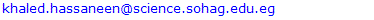Abstract

We study the single-particle (sp) properties of asymmetric nuclear within the framework of the Brueckner-Hartree-Fock (BHF) approach extended by including a phenomenological three-body force (3BF). These properties are the single-particle energy, the nucleon effective masses and the nuclear symmetry potential. Two kinds of realistic nucleon-nucleon interactions have been extensively used in the present BHF calculations. One is the charge-dependent Bonn potential (CD-Bonn) and the other is local soft core Argonne (V18). It is demonstrated that the single-particle potential becomes more attractive for the proton and more repulsive for the neutron at higher asymmetries. Also, the symmetry potentials at the normal density for different asymmetry parameters are in good agreement with the empirical value constrained by the experimental data. Finally, the neutron and proton effective masses show strong isospin splitting with m*n > m*p on the whole range of asymmetry parameters. So the 3BF force has effects turn out to be crucial for predicting reliably the sp properties within the Brueckner framework.

Keywords: Asymmetric Nuclear Matter, Single-Particle Potential, Effective mass, Nuclear Symmetry Potential

Cite this paper: Khaled S. A. Hassaneen, Alaa M. El-Sheikh, Hoda M. Abou-Elsebaa, Single-particle Properties of Asymmetric Nuclear Matter Based on Two- and Three-Body Forces within Brueckner Theory, Journal of Nuclear and Particle Physics, Vol. 11 No. 2, 2021, pp. 38-46. doi: 10.5923/j.jnpp.20211102.02.

### 1. Introduction

One of the fundamental points of nuclear physics and nuclear astrophysics is to study the single particle (sp) properties of asymmetric nuclear matter in a wide range of density. The momentum dependence of the single-particle (sp) potential in nuclear medium is described by nucleon effective mass. The nucleon effective masses assumes a significant part in understanding many intriguing physical quantities in nuclear physics and astrophysics , like the properties of nucleon superfluidity in nuclear matter , nucleon-nucleon (NN) cross sections in thick nuclear matter  and the dynamical advancement of heavy-ion collisions (HIC) at intermediate energies .
The determination of the momentum-dependence of symmetry potential and the neutron-proton effective mass splitting in neutron-rich nuclear matter is receiving more and more attention . These properties are depend on the structure of asymmetric nuclear matter at densities around and below the nuclear saturation density. The properties of asymmetric nuclear matter can be anticipated by embracing different nuclear many-body approaches, including phenomenological methods and microscopic approaches.
It is well known that the non-relativistic approaches using realistic two-body NN interactions are not reproduce the correct saturation point of symmetric nuclear matter, and three-body forces (3BF) are required . In recent years, the sp properties of asymmetric nuclear matter have been investigated extensively within the framework of various microscopic approaches including the Brueckner-Hartree-Fock (BHF), and the extended BHF approaches [7,8], the relativistic Dirac-BHF (DBHF) theory , the in-medium T-matrix and Green function methods , and the many- body variational approach . There are two kinds of 3BF, the phenomenological 3BF such as the Urbana 3BF  and the microscopic one .
The paper is arranged as follows. In the next section we discuss the BHF approach of asymmetric nuclear matter, then we extend it to include the 3BF rearrangement contribution in calculating sp properties in asymmetric nuclear matter. Our numerical results are reported in Sec. III, we will concentrate on the discussion of the effects of the 3BF rearrangement on neutron and proton sp properties including the sp potentials, the isospin splitting of the effective mass and the density dependence of the symmetry potential, based on the BHF approximation and BHF with the inclusion of phenomenological 3BF using two realistic nucleon-nucleon potentials are the CD-Bonn potential and Argonne (V18) potential. Finally, we summarize our present investigation in Sec. IV.

### 2. The BHF Approach of Asymmetric Matter

The BHF approach of asymmetric nuclear matter [14-18] starts with the construction of all the G matrices describing the effective interaction between two nucleons in the presence of a surrounding medium. They are obtained by solving the well-known Bethe-Goldstone equation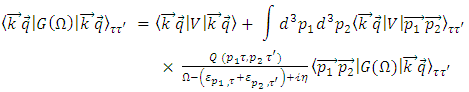(1)
where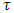= n,p indicates the isospin projection of the two nucleons in the initial, intermediate and final states, V denotes the bare NN interaction,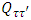the Pauli operator that allows only intermediate states compatible with the Pauli principle, and ω, the so-called starting energy, corresponds to the sum of non-relativistic energies of the interacting nucleons. The single-particle energy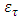of a nucleon with momentum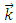is given by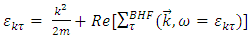(2)
The single-particle potential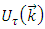or self-energy of a nucleon with isospin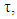represents the mean field "felt" by a nucleon due to its interaction with the other nucleons of the medium. In the BHF approach,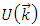is calculated through the "on-shell energy" G-matrix, and is given by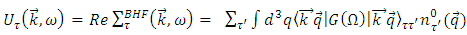(3)
where the sum runs over all neutron and proton occupied states and where the matrix elements are properly anti-symmetrized. In this equation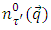refers to the occupation probability of a free Fermi gas of protons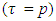and neutrons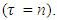This means for asymmetric matter with a total density ρ and asymmetry α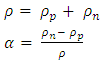(4)
this occupation probability is defined by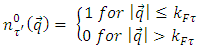(5)
with Fermi momenta for protons (kFp) and neutrons (kFn) which are related to the corresponding densities by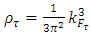(6)
In solving the Bethe-Goldstone equation for the G-matrix, we adopt the continuous choice for the single-particle potential Uτ(k) since it has been proved to provide much faster convergence of the hole-line expansion for the energy per nucleon of nuclear matter up to high densities than the gap choice [19,20]. Once a self-consistent solution of Eqs. (1) and (3) is achieved, the energy per particle can be calculated as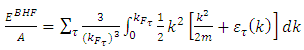(7)
The BHF calculation carried out in this work uses the realistic nucleon-nucleon interaction as two-body force (2BF) supplemented with a three-body force of Urbana type  which for the use in BHF calculations was reduced to a two-body density dependent force by averaging over the third nucleon in the medium . This three-body force contains two parameters that are fixed by requiring that the BHF calculation reproduces the energy and saturation density of symmetric nuclear matter. Explicitly, the 3BF is written as the sum of two terms: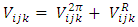(8)
where the first one is an attractive term, due to two-pion exchange with excitation of an intermediate Δ-resonance, and the second one is a repulsive phenomenological central term. The two-pion exchange contribution is a cyclic sum over the nucleon indices i, j, k of products of anticommutator {,} and commutator [,] terms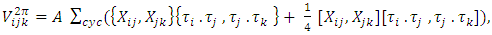(9)
where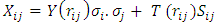(10)
is the one- pion exchange operator, σ and τ are the Pauli spin and isospin operators, and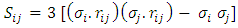is the tensor operator. Y(r) and T(r) are the Yukawa and tensor functions, respectively, associated to the one- pion exchange, as in the two {body potential. The repulsive part is taken as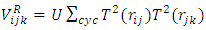(11)
The constants A and U in the previous equations can be adjusted to reproduce observed nuclear properties . When 3BF are included in BHF calculations, these enter at two levels. First, a density-dependent effective two-body interaction is added to the bare nucleon-nucleon interaction in a standard G-matrix calculation. In addition, the total energy has to be corrected to avoid double counting of the 3BF contribution [23,24]. At the lowest order this can be achieved by subtracting the Hartree-Fock contribution due to 3BF only :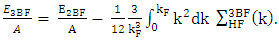(12)
We stress that the Hartree-Fock self-energy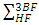coming from the 3BF is calculated from an effective two nucleons potential at the lowest order, in keeping with the procedure established Ref. .
Regarding Un/p as functions of the asymmetry parameter α, one can easily verify that the following approximate relation applies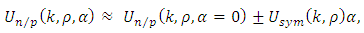(13)
with the ± referring to neutron/proton, respectively. The difference between the neutron and proton potentials then gives an accurate estimate for the strength of the isovector or symmetry potential in asymmetric nuclear matter, i.e.,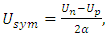(14)
which is of particular interest and importance for nuclear reactions induced by neutron-rich nuclei.
Another quantity which characterizes the single-particle potential is the effective mass. It is a measure of the nonlocal nature of the single-particle potential felt by a nucleon in a strongly interacting nuclear medium. It describes the momentum dependence of the single-particle potential and is evaluated from the slope of U at the Fermi momentum. Combining Eqs. 2 and 3 can easily yield the following expression of effective mass,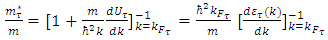(15)

### 3. Results and Discussion

As a first step we would like to discuss the single-particle potentials or the real part of self-energies as a function of the asymmetry parameter of nuclear matter α. For that purpose we consider nuclear matter at the empirical value for the saturation density of symmetric nuclear matter (ρ = 0.16 fm-3) and consider the self-energies for various values of the asymmetry parameter α. All the results discussed in this work have been computed for the charge-dependent Bonn (CD-Bonn) potential, defined in , which is nonlocal and exhibits a softer tensor component compared to another realistic nucleon-nucleon potential such as the Argonne V18 , which are local. As well, it represents a phenomenological parameterization in real space, including 18 spin-isospin operators. It is a typical example of a particularly strong, but finite, short-range core.
In order to discuss clearly the 3BF effects, firstly, we compare in Figure 1. the single-particle potentials or real-part of the on-shell self-energies in symmetric nuclear matter for both approaches obtained in four different densities ranging from 0.08, 0.16, 0.32 to 0.48 fm-3. In the case of CD-Bonn interaction, it is seen that the single-particle potential at the BHF level (solid curves) without including any 3BF is most attractive. At low densities below and around the normal nuclear matter density, the 3BF effects (red dashed curves) are reasonably small.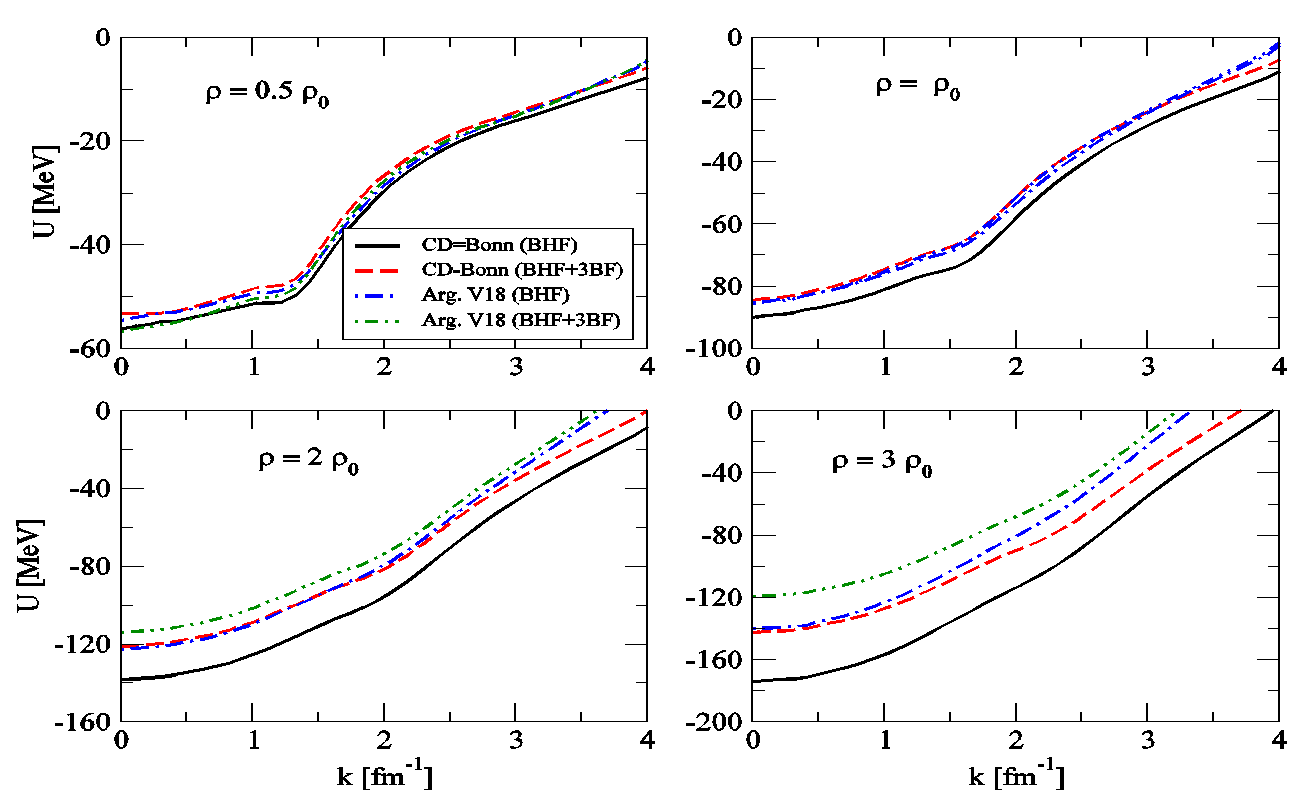Figure 1. Single-particle potentials calculated in symmetric nuclear matter within the BHF approach with and without 3BF forces and given by Eq. (3). Results are carried out using both the CD-Bonn and Argonne V18 interactions at different values of typical densities ρ
The 3BF effects turn out to become significant rapidly as the density increases. In particular, the depth of the potential, i.e. U0 (k = 0), reduces from -56.26 to -53.06 MeV at ρ = 0.08 fm-3, whereas at the normal density ρ0, i.e., at ρ = 0.16 fm-3, it begins from -90.02 and reduces to -84.51 MeV. This difference increases at high density, where the depth has the value -138.41 MeV and becomes -118.80 MeV when the 3BF included in BHF approach at density ρ = 0.32 fm-3.
As well, when the density has three times of the normal density ρ0, i.e. ρ = 0.48 fm-3 = 3 ρ0, the depth decreases from -173.83 to -142.52 MeV. This means that within the framework of the Brueckner theory, the 3BF is expected to affect the predicted single-particle potential or self-energies in two different ways: first, it influences the self-energies at the BHF and the extended BHF levels directly via its modification of the G-matrix. This means that, the full single-particle potential contains two parts, i.e., U (k) = UBHF (k) + U3BF (k), where UBHF (k) corresponds to the lowest-order BHF single-particle potential; whereas U3BF (k) is the contribution induced by the 3BF. Secondly, it may induce a strong contribution as a repulsion to the single-particle potential especially at high densities. Finally we can decide that the 3BF effect via its modification of the G-matrix at the BHF level provides a moderate repulsion at high densities which is more pronounced at lower momenta and weakens the momentum dependence of the single-particle potential.
In the case of Argonne V18 interaction, one finds a different scenario: Argonne V18 has a harder short-range core compared to CD-Bonn interaction and thus leads, in general, to a repulsive nature for the on-shell self-energy, not only at low or near to the normal density ρ0 but for all higher densities in the case of the BHF level. This behavior is observed in detail in Figure 1. where that results for single-particle potential shown by the dotted dashed lines. One finds that the Argonne V18 interaction yields much less attractive self-energies, the values of the self-energies at k=0, are -54.63, -85.34 , -122.79 and -140.20 MeV, compared to resulted by CD-Bonn interaction. This is already a first indication for the differences between the two interactions considered, the local interaction Argonne V18 is stiffer than the nonlocal CD-Bonn potential. Also, one can see from Figure 1. that the BHF self-energies do not have a simple parabolic shape as a function of the momentum.
In contrary to the CD-Bonn interaction, when the 3BF included in the G-matrix with Argonne V18 interaction at the BHF level as an effective interaction (dash-double dotted lines), the situation is different. Compared to the BHF results, the BHF plus 3BF does not shows any an additional repulsion for the single-particle potentials especially at low densities. Also, at normal density, the 3BF effect is relatively small. Whereas inclusion of the 3BF effect via the G-matrix alone provides a moderate repulsion at high densities and shifts up the potential in the whole momentum region. As well, this repulsion is more pronounced at lower momenta and thus weakens the momentum dependence of the single-particle potential.
In general, we can say that compared to the BHF results, the BHF plus 3BF exhibits an additional repulsion for the single-particle potentials. This repulsion in effective three-body interaction may be induced from the effects of sub-nucleonic degrees of freedom, like, e.g., the many-body effects arising from Δ excitations of the nucleons [12,13]. As a consequence, it has been shown in Refs. [29,30] such a strongly repulsive and momentum-dependent contribution induced by the 3BF is crucial for reducing the disagreement of the large-density and high-momentum BHF single-particle potential in symmetric matter with the parameterized potential for describing elliptic flow data  and those predicted by the Dirac Brueckner-Hartree-Fock approach .
For asymmetric nuclear matter or neutron-rich nuclear matter, the single-particle potential can be split into two components that are called the neutron and proton single-particle potentials. Moreover, as shown in of Figure 2. the on-shell values of them are presented as a function of the momentum, for various values of the asymmetry parameter α ranging from 0.2 to 0.8. Both the CD-Bonn and the Argonne V18 interactions have been used to generate the results for this figure at fixed density ρ = 0.16 fm-3 which agrees with kF = 1.333 fm-1 of symmetric nuclear matter. As expected, the BHF single-particle potentials for protons are more attractive than those for neutrons and that the depth of the potential for the protons is more attractive as the neutron excess α increases. Also its momentum dependence is too weak at large densities and high momenta for describing the high-energy elliptic flow data. This reflects the fact that the effective interaction is more attractive between protons and neutrons than between nucleons of the same isospin. This means that the SD tensor interaction felt by a neutron from the surrounding protons is weaker than that felt by a proton from the surrounding neutrons [14,15]. Also, from Figure 1. in the case of Argonne V18 interaction, it is seen that at asymmetry parameter α = 0.2, the 3BF effect is negligibly weak, while it gives an overall repulsive contribution to the proton and neutron at high values of α.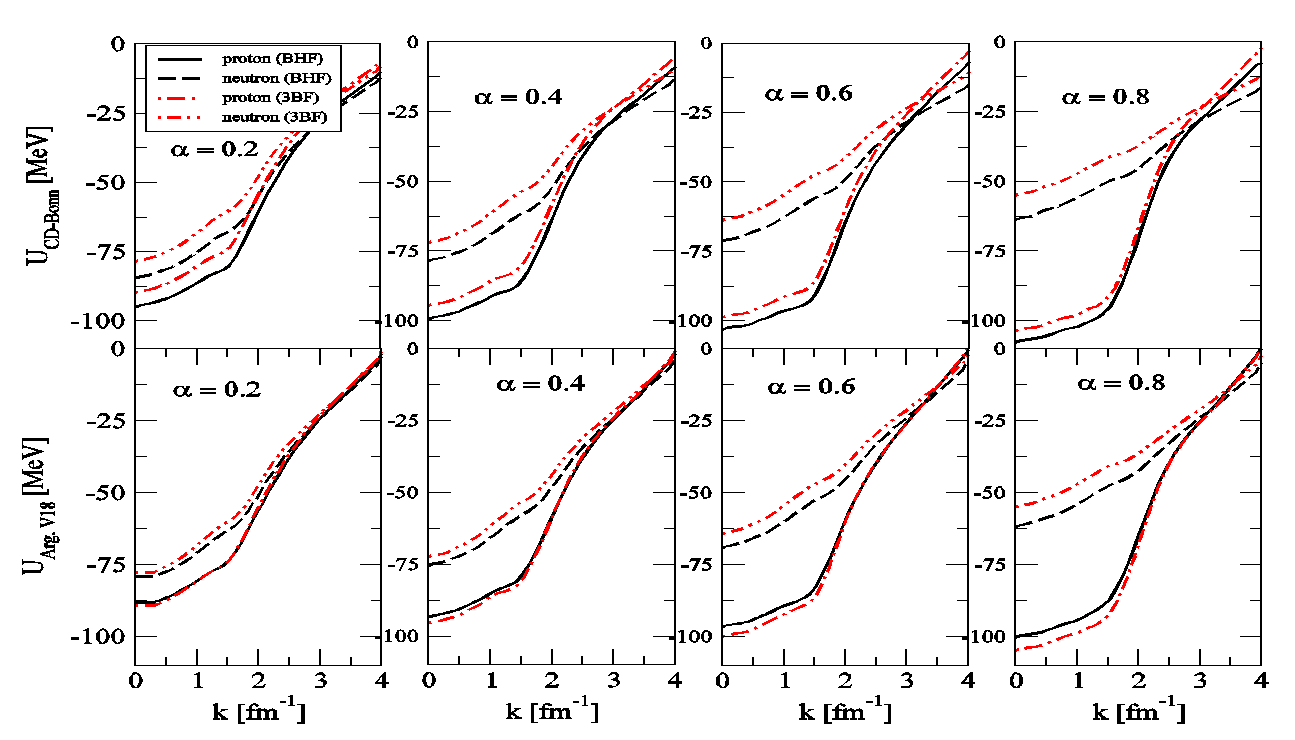Figure 2. Single-particle potentials calculated within the BHF approach with and without 3BF forces and given by Eq. (3). Results are carried out using both the CD-Bonn and Argonne V18 interactions at density ρ = 0.16 fm-3 for different values of asymmetry parameter α
In order to concentrate on only the isospin dependence, we present in Figure 3. the BHF (black lines) and BHF+3BF (red lines) single-particle potentials with respect to the asymmetry parameter α at the momentum k = 0 fm-1, i.e., the single-particle potential depth. It can be seen clearly that with and without 3BF contributions for proton and neutron potential depths versus asymmetries both have the distinct linear behavior when the two different interactions are considered, i.e., CD-Bonn (left panel) and Argonne V18 (right panel). Going from symmetric (α = 0) to near of neutron matter (α = 0.8), the proton single-particle potential for two cases is significantly decreased and until disappears as a consequence of the reduced number of the protons.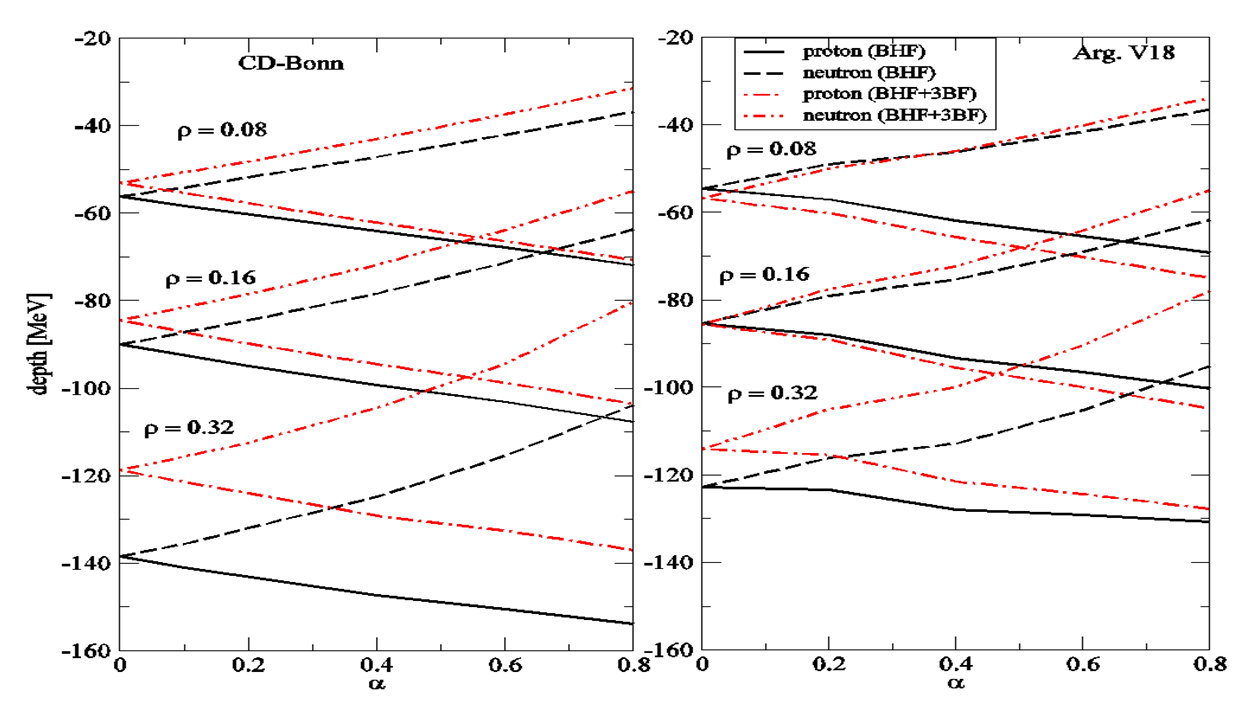Figure 3. The proton and neutron single-particle potentials as a function of the asymmetry parameter α at various densities and momentum equal to zero, i.e. potential depth
As discussed in Ref. , the above predicted different behavior of proton and neutron potentials or self-energies versus asymmetry parameter α is mainly caused by the isospin-singlet T = 0 SD tensor component of the nucleon-nucleon interaction and is readily understood as follows. According to the experimental data on the phase shifts of nucleon-nucleon scattering, the SD channel is strongly attractive at low energies. As the neutron excess increases, the attraction of the SD interaction between two unlike nucleons becomes stronger for protons and weaker for neutrons at relatively low momenta. The isospin T = 1 channel contribution is associated with the variations of the neutron and proton Fermi surfaces in neutron-rich matter. Its effect on the splitting of the neutron and proton potentials is opposite and much smaller as compared with the SD channel contribution at low momenta. The attraction of the SD channel decreases with energy, so that for high enough momenta, the splitting of the proton and neutron potentials in neutron- rich matter may vanish and even become opposite due to the competition between the T = 0 and T = 1 channel effects.
The results for the isospin symmetry potential which describes the difference between the neutron and proton sp potentials in neutron-rich matter as a function of the nucleon kinetic energy at the normal density ρ = 0.16fm-3 for different asymmetry parameters α are shown in Figure 4. it is calculated using Eq. 14. The closed gray area is the empirical information from Lane potential , we compare our results with the Dirac Brueckner Hartree Fock (DBHF) approach using Bonn B potential .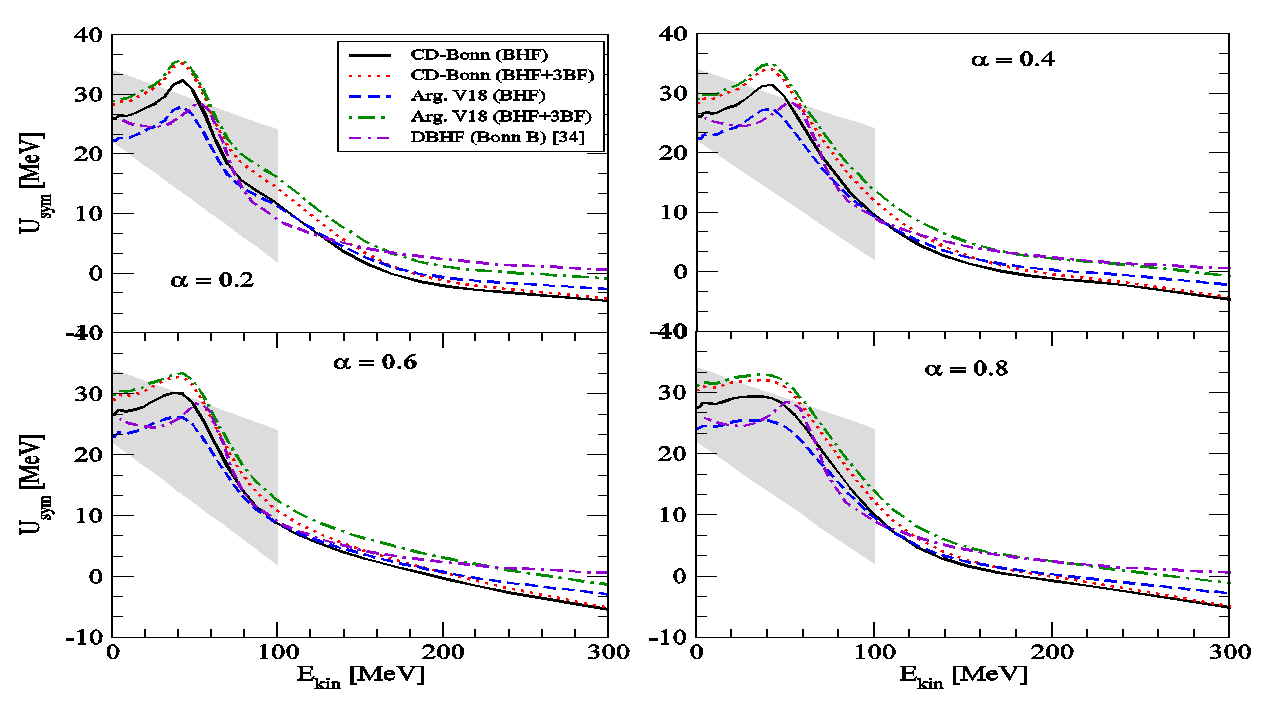Figure 4. The isospin symmetry potential as a function of the nucleon kinetic energy at ρ = 0.16 fm-3 for different α using both the CD-Bonn and Argonne V18 interactions. The closed gray area is the empirical information from Lane potential . The results are compared with the Dirac Brueckner Hartree Fock (DBHF) approach using Bonn B potential 
The results increase with increasing Ekin until it reach a maximum value then it decrease with increasing Ekin. The differences between the curves be more apparent at low Ekin. It is seen that when using the Argonne V18 potential at the BHF without including any 3BF has low results for the isospin symmetry potential for all kinetic energies, the 3BF effects make the results more repulsion. We compared our results with the empirical information from Lane potential, it is seen that the isospin symmetry potentials at the normal density for different asymmetry parameters are in good agreement with the empirical information from Lane potential. Our results which are consistent with the empirical information and the DBHF results.
The nucleon effective mass m*/m is of great interest in nuclear physics and nuclear astrophysics. It describes the momentum dependence of the obtained single particle potential in nuclear matter. We used the Eq. (15) to obtain the effective mass. The effective mass can be evaluated from the slope of the single particle potential.
We calculate the nucleon effective mass for symmetric nuclear matter at the Fermi momentum k = kF as a function of density ρ, it is shown in Figure 5. The results are carried out within the BHF with and without 3BF using both the CD-Bonn and Argonne V18 interactions, CD-Bonn potential without 3BF (black solid line) with 3BF (red dotted line), Argonne V18 potential without 3BF (green dashed line) with 3BF (blue dashed-dotted line). When using the BHF approximation only the results are decrease with increasing density, begin with 0.85 at small density until it reach 0.5 at density approximately equal to 0.7 fm-3, for both the CD-Bonn and Argonne V18 interactions the results are close to each other for different values of ρ.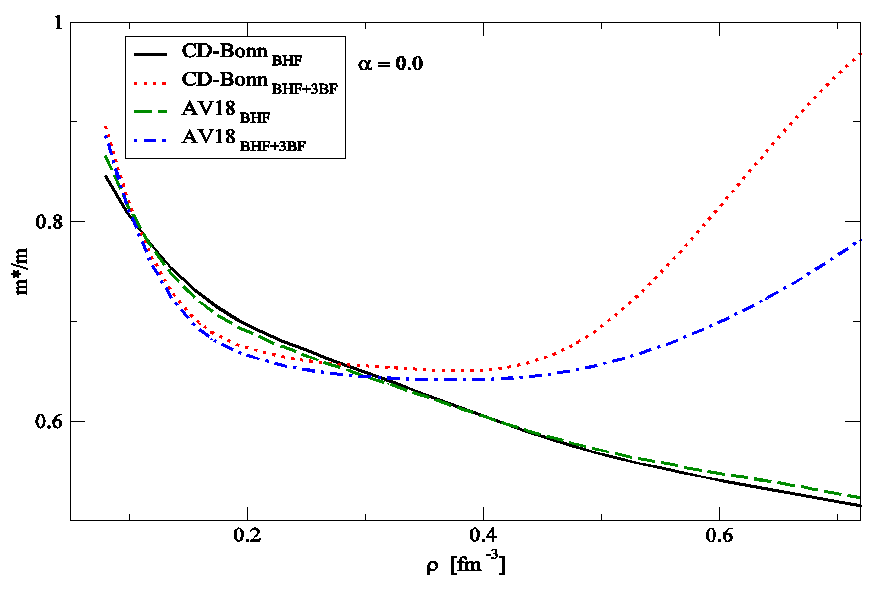Figure 5. The nucleon effective mass for symmetric nuclear matter as a function of density ρ in fm-3. The results are carried out within the BHF with and without 3BF using both the CD-Bonn and Argonne
However when we using the BHF approximation with the inclusion of 3BF the values of the effective masses first decrease with increasing density then it begin to increase with increasing density, at low density the values of the two potentials are close to each other’s while at high density the values of the effective masses for CD-Bonn potential are greater than the values of the Argonne V18 potential. At density equal to 0.7 fm-3 the value of the effective mass reach to 0.95 for CD-Bonn potential.
The nucleon effective mass for asymmetric nuclear matter as a function of density ρ in fm-3 at different asymmetry parameter α is displayed in Figure 6. using the CD-Bonn potential, left panel for proton, right panel for neutron, the BHF (black lines) and BHF+3BF (red lines). It is clear from Figure 6. that the values of the effective mass for proton always less than that for neutron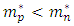on the whole range of asymmetry parameters.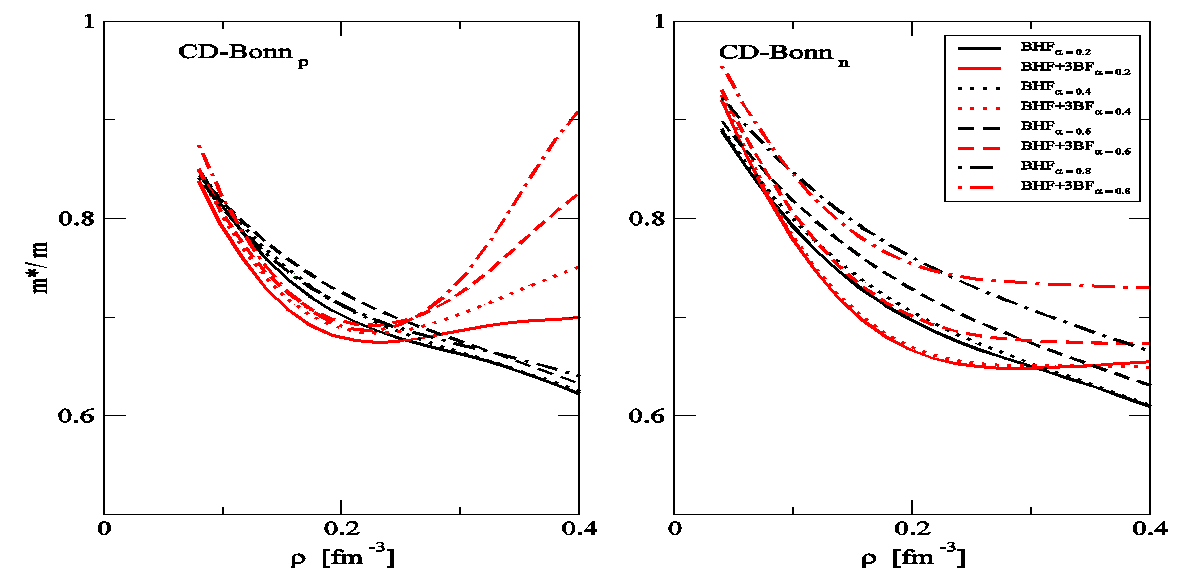Figure 6. Proton (left- panel) and neutron (right- panel) effective masses as a function of the total density ρ in fm-3 using CD-Bonn potential within BHF (black lines) and BHF+3BF (red lines) approaches at different values of the asymmetry parameter α = 0.2 (solid lines), 0.4 (dotted lines), 0.6 (short dashes lines) and 0.8 (dot- dashed lines)
It is seen that the results for the effective mass increase with increasing the asymmetry parameter α. In the left panel, when we using the BHF approximation only the results begin with 0.85 at ρ = 0.1 fm-3 then it decrease until it reach 0.62 at density equal to 0.4 fm-3. The inclusion of 3BF leads to a significant and global enhancement of the effective mass at density above 0.2 fm-3. The 3BF effect is to increase significantly the effective mass at large densities due to its strongly momentum-dependent contribution to the sp potential. In the right panel, the effect of the 3BF at high densities is lower than that compared to the proton.
In Figure 7. the same as Figure 6. but using Argonne V18 potential. When we use the 3BF effect the results for the effective mass decreases at low densities below and around the saturation density and its density dependence becomes quite weak, the effective mass is larger than the BHF only, strong effect of the 3BF at high densities especially for proton more than the neutron.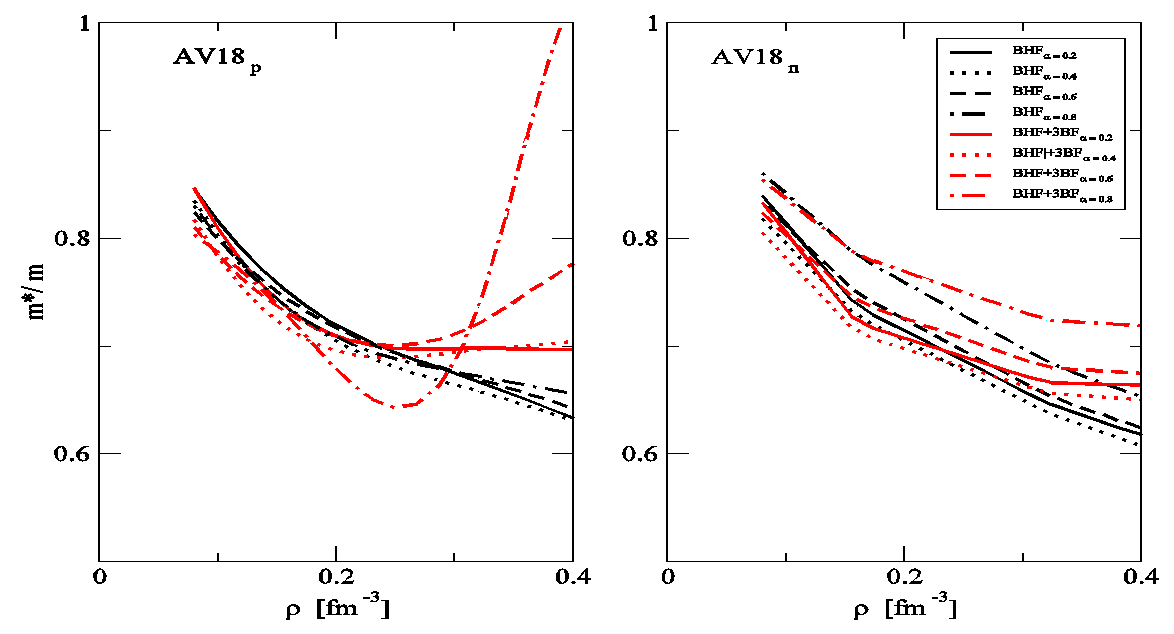Figure 7. The same as Figure 7. but using Argonne V18 potential

### 4. Conclusions

The sp properties of asymmetric nuclear matter within the BHF approach with and without the inclusion of 3BF are studied. It is found that the 3BF make the results of the single-particle potential more repulsion than BHF only and the Argonne V18 potential is more repulsive than CD-Bonn potential. It is demonstrated that the single-particle potential becomes more attractive for the proton and more repulsive for the neutron at higher values of α. The isospin symmetry potentials at the normal density for different α is calculated, the results are in good agreement with the empirical information from Lane potential. We have predicted the nucleon effective masses and their isospin dependence in asymmetric nuclear matter, at low densities, the 3BF effect is fairly small. Also, the neutron and proton effective masses show strong isospin splitting with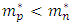on the whole range of asymmetry parameters.

### ACKNOWLEDGEMENTS

One of the authors, Khaled S. A. Hassaneen, would like to thank Professors H. Müther for helpful discussions and guidance as well as Professor M. Baldo for providing three-body force code.

### References

  D. Lunney, J. M. Pearson and C. Thibault, Rev. Mod. Phys. 75, 1021 (2003).  U. Lombardo, P. Schuck and W. Zuo, Phys. Rev. C 64, 021301 (2001).  G. Giansiracusa, U. Lombardo and N. Sandulescu, Phys. Rev. C 53, R1478 (1996).  B. A. Li, L. W. Chen and C. M. Ko, Phys. Rep. 464, 113 (2008).  C. B. Das, S. Das Gupta, C. Gale and B. A. LI, Phys. Rev. C 67, 034611 (2003).  H. M. Abou-Elsebaa, E. M. Darwish, and Kh. S. A. Hassaneen, Moscow University Physics Bulletin, Vol. 75, No. 4, 320 (2020).  I. Bombaci, U. Lombardo, Phys. Rev. C 44, 1892 (1991).  W. Zuo, U. Lombardo, H.J. Schulze, Z.H. Li, Phys. Rev. C 74, 014317 (2006).  T. Klahn, D. Blaschke, S. Typel et al., Phys. Rev. C 74, 035802 (2006).  T. Frick, H. Müther, A. Rios, A. Polls, A. Ramos, Phys. Rev. C 71, 014313 (2005).  R.B. Wiringa, V. Fiks, A. Fabrocini, Phys. Rev. C 38, 1010 (1988).  J. Carlson, V.R. Pandharipande, R.B. Wiringa, Nucl. Phys. A 401, 59 (1983).  P. Grangé, A. Lejeune, M. Martzolff, and J. F. Mathiot, Phys. Rev. C 40, 1040 (1989).  W. Zuo, I. Bombaci and U. Lombardo, Phys. Rev. C 60, 024605 (1999).  Kh. S. A. Hassaneen and H. Müther, Phys. Rev. C 70, 054308 (2004).  K. Gad and Kh. S. A. Hassaneen, Nucl. Phys. A 793, 67 (2007).  Khaled Hassaneen and Khalaf Gad, J. Phys. Soc. Jpn. 77, 084201 (2008).  P. Gögelein, E. N. E. van Dalen, Kh. Gad, Kh. S. A. Hassaneen, and H. Müther, Phys. Rev. C 79, 024308 (2009).  M. Baldo, A. Fiasconaro, H. Q. SONGQ, G. Giansiracusa, and U. Lombardo, Phys. Rev. C 65, 017303 (2002).  Kh.S.A. Hassaneen, H.M. Abo-Elsebaa, E.A. Sultan, and H.M.M. Mansour, Ann. Phys. (N.Y.) 326, 566 (2011).  M. Baldo and L. Ferreira, Phys. Rev. C 59, 682 (1999).  Private communication with Professor M. Baldo.  K. Hebeler, A. Schwenk, Phys. Rev. C 82, 014314 (2010).  A. Carbone, A. Polls, A. Rios, Phys. Rev. C 88, 044302 (2013).  Hugo F. Arellano, Felipe Isaule, and Arnau Rios, Eur. Phys. J. A 52: 299 (2016).  Hesham Mansour, Khalaf Gad, and Khaled S.A. Hassaneen, Prog. Theor. Phys. 123, 687 (2010).  R. Machleidt, F. Sammarruca, and Y. Song, Phys. Rev. C 53, R1483 (1996).  R. B. Wiringa, V. G. J. Stoks and R. Schiavilla, Phys. Rev. C 51, 38 (1995).  W. Zuo, L. G. Cao, B. A. Li, U. Lombardo and C. W. Shen, Phys. Rev. C 72, 014005 (2005).  W. Zuo, U. Lombardo, H. J. Schulze and Z. H. Li, Phys. Rev. C 74, 014317 (2006).  P. Danielewicz, Nucl. Phys. A 673, 375 (2000).  E. N. E. van Dalen, C. Fuchs and A. Faessler, Phys. Rev. Lett. 95, 022302 (2005).  A. M. Lane, Nucl. Phys. 35, 676 (1962).  F. Sammarruca, International Journal of Modern Physics E, Vol. 19, No. 7 (2010) 1259–1313.